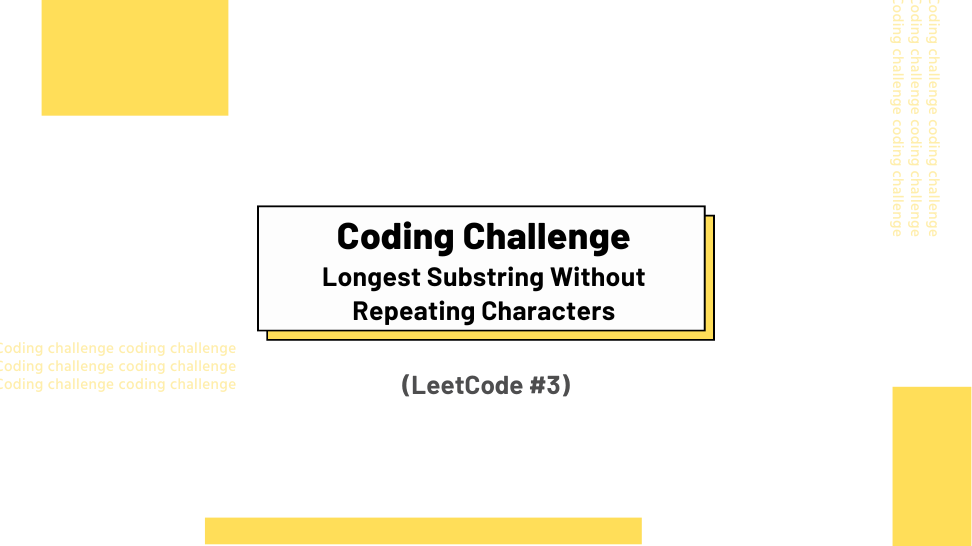## Challenge Statement

• Given a string s, find the length of the longest substring without repeating characters.
• This challenge corresponds to LeetCode #3.

### Constraints

• `0 <= s.length <= 5 * 104`
• s consists of English letters, digits, symbols, and spaces.

### Example 1:

Input: `s = "abcabcbb"`

Output: 3

Explanation: The answer is “abc”, with a length of 3.

### Example 2:

Input: `s = "bbbbb"`

Output: 1

Explanation: The answer is “b”, with the length of 1.

### Example 3:

Input: `s = "pwwkew"`

Output: 3

Explanation: The answer is “wke”, with a length of 3.

Notice that the answer must be a substring, “pwke” is a subsequence and not a substring.

## Solution

Below is my solution and some test cases. The solution has a linear time complexity of O(n) and constant space complexity O(1), where n is the number of characters in the input string.